Introduction To Electric Circuits - 9 Edition - Chapter 7.5 - Problem P7.5-1
Register Now

Join StudySoup

Get Full Access to Introduction To Electric Circuits - 9 Edition - Chapter 7.5 - Problem P7.5-19781118477502

# Nikola Tesla (18571943) was an American electrical engineer who experimented with

Introduction to Electric Circuits | 9th Edition

Problem P7.5-1

Nikola Tesla (1857–1943) was an American electrical engineer who experimented with electric induction. Tesla built a large coil with a very large inductance, shown in Figure P 7.5-1. The coil was connected to a source current

$$i_{\mathrm{s}}=100 \sin 400 t\ \mathrm{A}$$

so that the inductor current $$i_L = i_s$$. Find the voltage across the inductor and explain the discharge in the air shown in the figure. Assume that L = 200 H and the average discharge distance is 2 m. Note that the dielectric strength of air is $$3 \times 10^6\ V/m$$.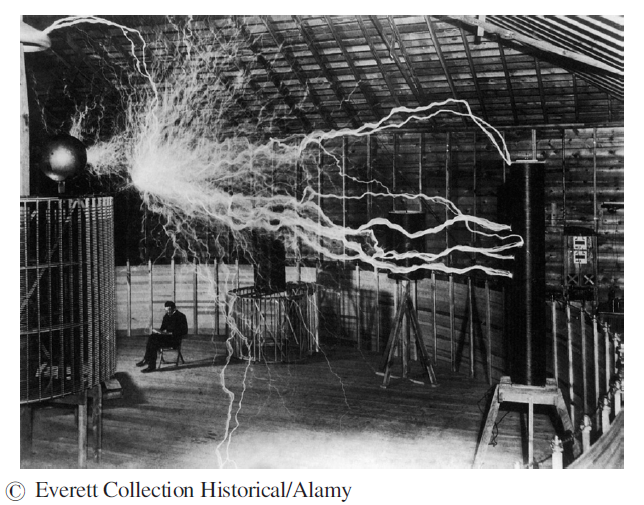Nikola Tesla sits impassively as alternating current induction coils discharge millions of volts with a roar audible 10 miles away (about 1910).

Accepted Solution
Step-by-Step Solution:

Step 1 of 3

It is required to find the voltage across the inductor. It can be found using the following formula: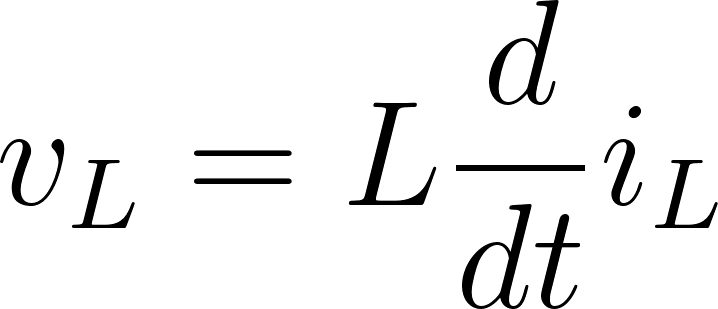For the given current and inductance we have: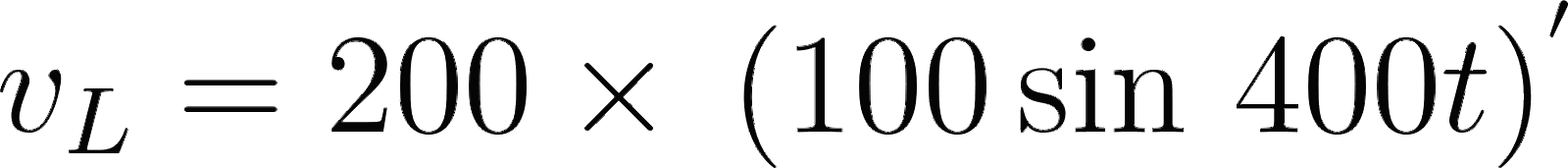=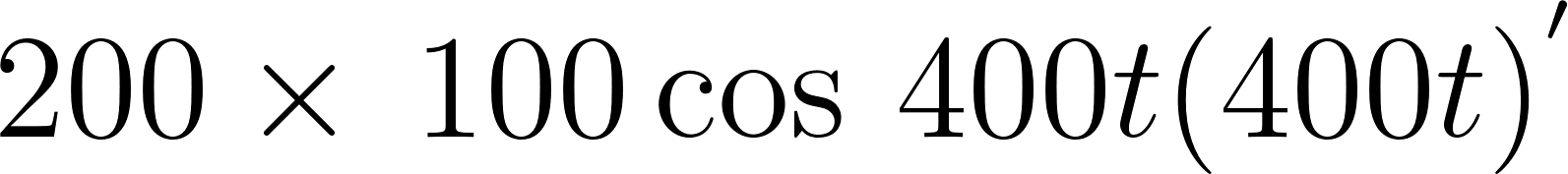==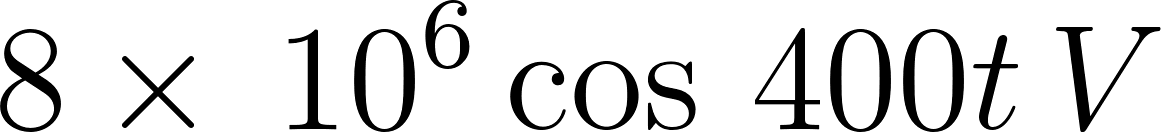=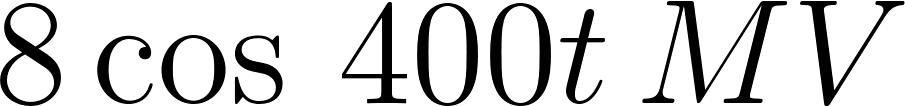###### Chapter 7.5, Problem P7.5-1 is Solved

Step 2 of 3

Step 3 of 3

Unlock Textbook Solution